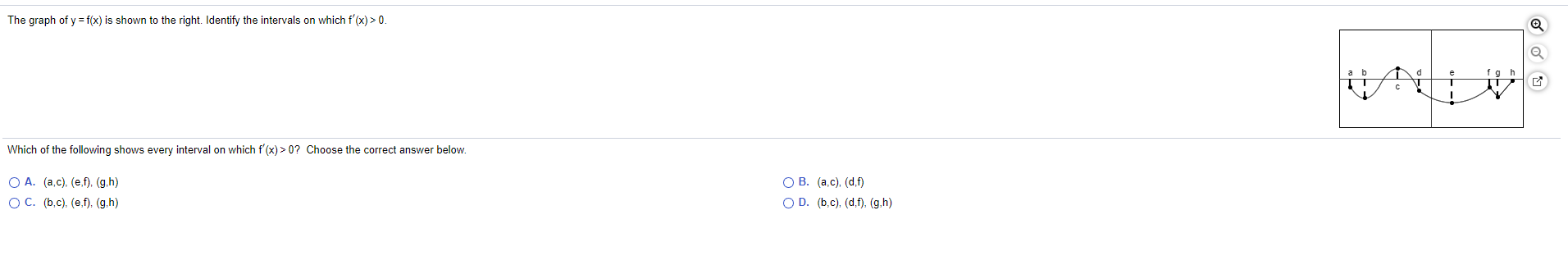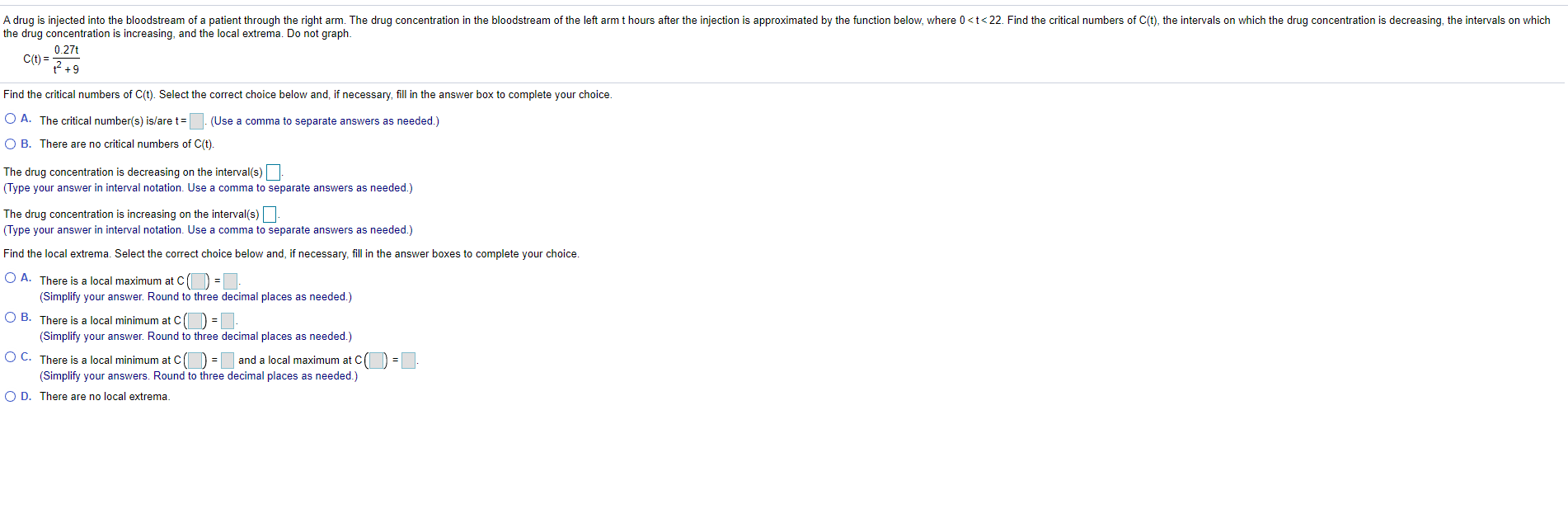### Create an Account

Already have account?

### Forgot Your Password ?

Home / Questions / The graph of y = f(x) is shown to the right. Identify the intervals on which f'(x) > 0. d ...

# The graph of y = f(x) is shown to the right. Identify the intervals on which f'(x) > 0. d fgh Which of the following shows every interval on which f'(x) > 0? Choose the correct answer below. O A. (a.c

The graph of y = f(x) is shown to the right. Identify the intervals on which f'(x) > 0. d fgh Which of the following shows every interval on which f'(x) > 0? Choose the correct answer below. O A. (a.c), (e,f), (g,h) OC. (b,c), (e,f), (g,h) OB. (ac), (d,f) OD. (b,c), (d.f), (g,h)
A drug is injected into the bloodstream of a patient through the right arm. The drug concentration in the bloodstream of the left arm t hours after the injection is approximated by the function below, where 0Apr 14 2021 View more View Less

#### Answer (Solved)Subscribe To Get Solution